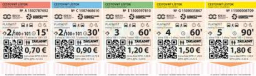# Tickets 11

There are 72 tickets to be shared and divided to Section X and Section Y in the ratio 4:5. How many tickets will Section Y receive?

Y =  40

### Step-by-step explanation:Did you find an error or inaccuracy? Feel free to write us. Thank you!

Tips for related online calculators
Need help calculating sum, simplifying, or multiplying fractions? Try our fraction calculator.
Check out our ratio calculator.
Do you want to perform natural numbers division - find the quotient and remainder?